Courses

# RS Aggarwal Test: Triangles

## 25 Questions MCQ Test Mathematics (Maths) Class 10 | RS Aggarwal Test: Triangles

Description
This mock test of RS Aggarwal Test: Triangles for UPSC helps you for every UPSC entrance exam. This contains 25 Multiple Choice Questions for UPSC RS Aggarwal Test: Triangles (mcq) to study with solutions a complete question bank. The solved questions answers in this RS Aggarwal Test: Triangles quiz give you a good mix of easy questions and tough questions. UPSC students definitely take this RS Aggarwal Test: Triangles exercise for a better result in the exam. You can find other RS Aggarwal Test: Triangles extra questions, long questions & short questions for UPSC on EduRev as well by searching above.
QUESTION: 1

### Triangle ABC is such that AB = 3 cm, BC = 2 cm and CA = 2.5 cm. Triangle DEF is similar to ΔABC. If EF = 4 cm, then the perimeter of ΔDEF is :

Solution: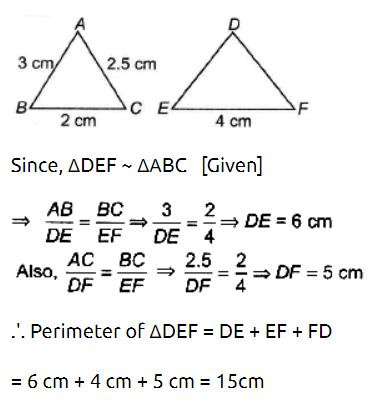QUESTION: 2

Solution:
QUESTION: 3

### In an equilateral triangle ABC, if AD ⊥ BC, then:

Solution:

Δ ABC is an equilateral triangle

By Pythagoras theorem in triangle ABD

AB2 = AD2 + BD2

but BD = 1/2 BC (∵ In a triangle, the perpendicular from the vertex to the base bisects the base)

thus AB2 = AD2 + {1/2 BC}2

AB2 = AD2 + 1/4 BC2

4 AB2 = 4AD2 + BC2

4 AB2 - BC2 = 4 AD2

(as AB = BC we can subtract them )

Thus 3AB2 = 4AD2

QUESTION: 4

ABC is a triangle and DE is drawn parallel to BC cutting the other sides at D and E. If AB = 3.6 cm, AC = 2.4 cm and AD = 2.1 cm, then AE is equal to :

Solution:
QUESTION: 5

The line segments joining the mid points of the sides of a triangle form four triangles each of which is :

Solution:

Given :△ABC, D, E and F are mid points of AB, BC, CA respectively.
Using mid point theorem we prove that □ADEF, □DBEF and □DECF are parallelograms. The diagonal of a parallelogram divides the parallelogram into two congruent triangles. So all triangles are congruent to each other. And each small triangle is similar to the original triangle.

QUESTION: 6

In ΔABC and ΔDEF, ∠A = 50°, ∠B = 70°, ∠C = 60°, ∠D = 60°, ∠E = 70°, ∠F = 50°, then ΔABC is similar to:

Solution:
QUESTION: 7

D, E, F are the mid points of the sides BC, CA and AB respectively of ΔABC. Then ΔDEF is congruent to triangle

Solution:

The correct option is Option D.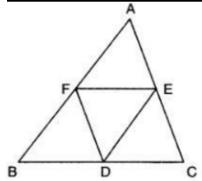Given : D, E and F are tge mid-point of the sides BC, CA and AB respectively of Δ ABC.

To prove : Δ DEF is congruent to traingle

Proof :

Since E and F are midpoints of AC and AB.

BC II FE and FE = ½ BC = BD (By mid point theorem)

BD II FE and BD = FE

Similarly, BF II DE and BF = DE

Hence, BDEF is a parallelogram (A pair of opposite sides are equal and parallel)

Similarly, we can prove that FDCE and AFDE are also parallelograms.

Now, BDEF is a parallelogram so it's diagonal FD divides it into two traingles of equal areas.

Therefore, ar(Δ BDF) = ar(Δ DEF).......... (i)

In parallelogram AFDE,

ar(Δ AFE) = ar(Δ DEF)   (EF is a diagonal)......... (ii)

In parallelogram FDCE,

ar(Δ CDE) = ar(Δ DEF)   (DE is a diagonal)...........(iii)

From (i), (ii) and (iii)

ar(Δ BDF) = ar(Δ AFE) = ar(Δ CDE) = ar(Δ DEF)..........(iv)

If area of traingles are equal then they are congruent.

Hence, Δ DEF is congruent to triangle Δ BDF = Δ AFE = Δ CDE.

QUESTION: 8

If in the triangles ABC and DEF, angle A is equal to angle E, both are equal to 40°, AB : ED = AC : EF and angle F is 65°, then angle B is :-

Solution:
QUESTION: 9

In a right angled ΔABC, right angled at A, if AD ⊥ BC such that AD = p, If BC = a, CA = b and AB = c, then: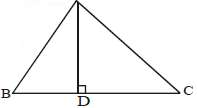Solution:

In ΔCAB and ΔADB
Angle B is common and Angle A=Angle D
So the triangles are similar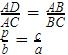a=cb/p
Now applying pythagoras theorem in
Δ ABC
H= P2+B2
BC2=AC2+AB2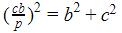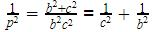QUESTION: 10

In the adjoining figure, XY is parallel to AC. If XY divides the triangle into equal parts, then the value of AX/AB  =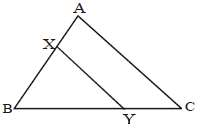Solution:
QUESTION: 11

The ratio of the corresponding sides of two similar triangles is 1 : 3. The ratio of their corresponding heights is :

Solution:
QUESTION: 12

The areas of two similar triangles are 49 cm2 and 64 cm2 respectively. The ratio of their corresponding sides is:

Solution:

If two triangles are similar, then the ratio of the area of both triangles is proportional to the square of the ratio of their corresponding sides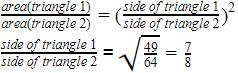QUESTION: 13

The areas of two similar triangles are 12 cm2 and 48 cm2. If the height of the smaller one is 2.1 cm, then the corresponding height of the bigger one is:

Solution:

Given,

Area of two similar triangles = 12 cm² , 48 cm² .

Area of bigger triangle = 48 cm²

Area of smaller triangle = 12 cm²

We know that, Ratio of the areas of two similar is equal to square of the ratio of corresponding heights or altitudes.

Given, Height of smaller triangle =2.1 cm.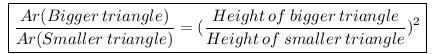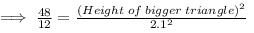4 = (Height of the triangle)² / 4.41

√(4 * 4.41) = Height of the bigger triangle

Height of the bigger triangle = 2 * 2.1 = 4.2 cm.

QUESTION: 14

In a triangle ABC, if AB, BC and AC are the three sides of the triangle, then which of the statements is necessarily true?

Solution:
QUESTION: 15

The sides of a triangle are 12 cm, 8 cm and 6 cm respectively, the triangle is :

Solution:
QUESTION: 16

In an equilateral triangle, the incentre, circumcentre, orthocentre and centroid are:

Solution:

The centroid is the intersection of the three medians while the incentre is the intersection of the three (internal) angle bisectors. In an equilateral triangle, each median is also an angle bisector (and vice versa), the centroid coincides with the incentre. In fact, the centroid, incentre, circumcentre and orthocentre of an equilateral triangle are coincide at the same point.

QUESTION: 17

In the adjoining figure D is the midpoint of BC of a  ΔABC. DM and DN are the perpendiculars on AB and AC respectively and DM = DN, then the ΔABC is :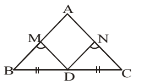Solution:
QUESTION: 18

Triangle ABC is such that AB = 9 cm, BC = 6 cm, AC = 7.5 cm. Triangle ΔDEF is similar to ABC, If EF = 12 cm then DE is :

Solution:
QUESTION: 19

In ΔABC, AB = 5 cm, AC = 7 cm. If AD is the angle bisector of ∠A. Then BD : CD is:

Solution:
QUESTION: 20

In a ΔABC, D is the mid-point of BC and E is mid-point of AD, BF passes through E. What is the ratio of AF : FC?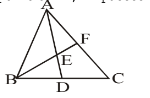Solution:

Construct DG || BF
In triangle ADG,
AE = ED ( given)
EF || DG ( by construction)
a line passing through the mid point of any side of a triangle, parallel to the other side, bisects the third side
So AF = FG    ...(1)
Now in triangle CBF
BD = DC ( given)
DG||BF ( by construction)
a line passing through the mid point of any side of a triangle, parallel to the other side, bisects the third side
So, FG = GC …(2)
Now, by (1) & (2)
AF = FG = GC
AF : FC = 1:2

QUESTION: 21

In the given figure, PS is the median then ∠QPS?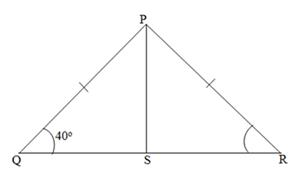Solution:

since PQ = PR

so ∠Q = ∠R = 40o

so ∠P comes out to be 100o

and since PS is a median, so it'll bisect the ∠P into two equal parts

so, ∠QPS = 50o

QUESTION: 22

The difference between altitude and base of a right angled triangle is 17 cm and its hypotenuse is 25 cm. What is the sum of the base and altitude of the triangle is ?

Solution:
QUESTION: 23

If AB, BC and AC be the three sides of a triangle ABC, which one of the following is true?

Solution:
QUESTION: 24

In the adjoining figure D, E and F are the mid-points of the sides BC, AC and AB respectively. ΔDEF is congruent to triangle :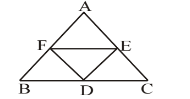Solution:

Given :△ABC, F, D and E are mid points of AB, BC, CA respectively.
Using mid point theorem we prove that □AEFD, □DBFF and □DCEF are parallelograms. The diagonal of a parallelogram divides the parallelogram into two congruent triangles. So all triangles are congruent to each other. So ΔDEF is congruent to
△AFE, △BFD and △CDE

QUESTION: 25

In the adjoining figure ∠BAC = 60° and BC = a, AC = b and AB = c, then :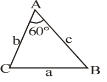Solution:

We have cosine formula as Cos A =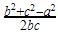So, Cos 60 =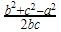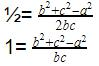b2+c2-a2=bc
a2=b2+c- bc